# Trigger Firing Sequence in Oracle

## Trigger Firing Sequence in Oracle with Examples

In this article, I am going to discuss Trigger Firing Sequence in Oracle with Examples. Please read our previous article where we discussed How to Create an Audit table using Triggers in Oracle with Examples.

##### Trigger Firing Sequence in Oracle

Let us assume that we have a table in the database and we created four different types of triggers. Suppose on the table we have created Before Statement Trigger, Before Row Trigger, After Row Trigger, and After Statement Trigger in the Oracle database. The firing sequence will be in the same order.

We should understand this firing sequence before we work on the triggers. Let us do an exercise on this firing sequence to get a better idea about the Firing of the Sequence of Oracle Triggers. In this exercise, we will be creating four triggers. The triggers are as follows.

1. Before Statement
2. Before Each Row
3. After Each Row
4. After Statement
##### Step 1: Create a Table in Oracle

Let us go ahead and create a table to work with triggers by executing the following CREATE table statement in the Oracle database.

```Create table test_emp
(
emp_id number,
First_name varchar2(100)
);
```

This table will have two columns EMP_ID and FIRST_NAME.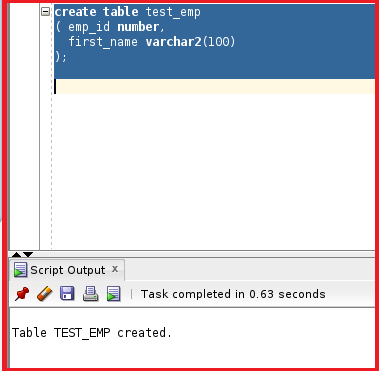So, the table TEST_EMP is created. Let us go ahead and create another table to store the sequence by executing the following CREATE Table Statement in the same Oracle database.

```Create table test_emp_sequence
(
seq number,
Trigger_type varchar2(100)
);
```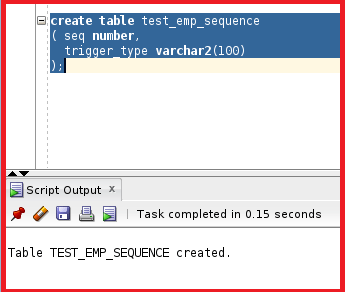So, the table TEST_EMP_SEQUENCE is also created. This table is used to store the firing sequence of the triggers. This table has two columns seq and trigger_type.

##### Step 2: Create Sequence S in Oracle

Let us go ahead and create a sequence by executing the following SQL Statement.

create sequence s;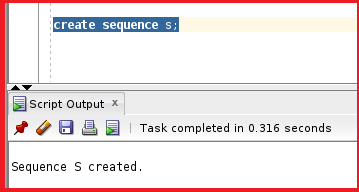##### Step 3: Creating Four Triggers in Oracle

We are going to create four triggers with BEFORE STATEMENT, BEFORE EACH ROW, AFTER EACH ROW, and AFTER STATEMENT.

##### Trigger1: Before Statement Trigger in Oracle

The following trigger is named with before_insert_stat where the timing is before and the event is insert. The trigger will insert the value of the sequence into the TEST_EMP_SEQUENCE table with the description as “before_insert_stat”.

```Create or replace trigger before_insert_stat
Before insert
On test_emp
Begin
Insert into test_emp_sequence values ( s.nextval,’before_insert_stat’);
End;
```

Let us go ahead and create the trigger.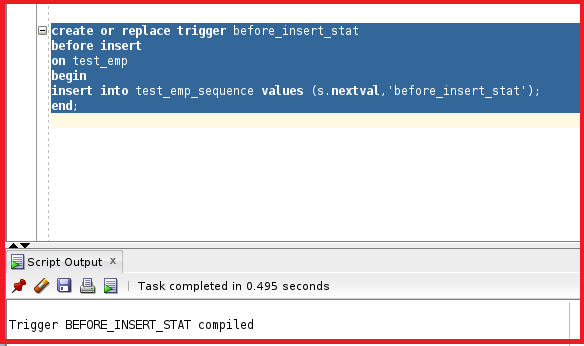So, the trigger is created. Let us go ahead and create the other triggers as well.

##### Trigger2: Before Each Row Trigger in Oracle

The following trigger is named with before_insert_each_row where the timing is before and the event is insert. The trigger will insert the value of the sequence for each row into the TEST_EMP_SEQUENCE table with the description as “before_insert_each_row”. So, if there are 3 rows then 3 sequences are inserted into the table TEST_EMP_SEQUENCE before the insert operation in the table TEST_EMP.

```Create or replace trigger before_insert_each_row
Before insert
On test_emp
For each row
Begin
Insert into test_emp_sequence values ( s.nextval,’before_insert_each_row’);
End;
```

Let us go ahead and create the trigger.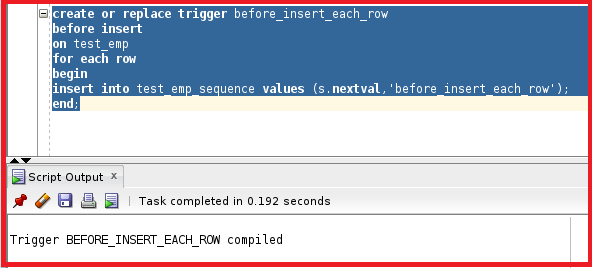The trigger is created. Let us go ahead and create the other two triggers.

##### Trigger3: After Each Row Trigger in Oracle

The following trigger is named After_insert_each_row where the timing is after and the event is insert. The trigger will insert the value of the sequence for each row into the TEST_EMP_SEQUENCE table with the description “after_insert_each_row”. So, if there are 3 rows and the insert operation is performed on TEST_EMP, then 3 sequences are inserted into the table TEST_EMP_SEQUENCE only after the insert operation is performed on the table TEST_EMP.

```Create or replace trigger after_insert_each_row
after insert
On test_emp
For each row
Begin
Insert into test_emp_sequence values ( s.nextval,’after_insert_each_row’);
End;
```

Let us go ahead and create the trigger.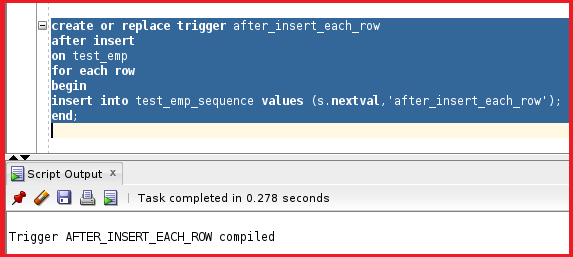##### Trigger4: After Statement Trigger in Oracle

The following trigger is named After_insert_stat where the timing is after and the event is insert. The trigger will insert the value of the sequence into the TEST_EMP_SEQUENCE table with the description “after_insert_stat”. So, if there are 3 rows and the insert operation is performed on TEST_EMP, then 3 sequences are inserted into the table TEST_EMP_SEQUENCE only after the insert operation is performed on the table TEST_EMP.

```Create or replace trigger after_insert_stat
after insert
On test_emp
For each row
Begin
Insert into test_emp_sequence values ( s.nextval,’after_insert_stat’);
End;
```

Let us go ahead and create the trigger.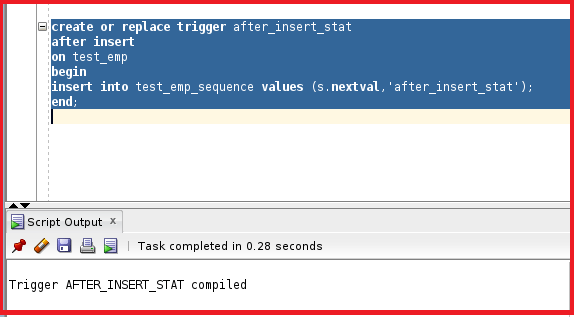The trigger is created successfully. So, all four triggers are created. Let us check the table details of the table TEST_EMP.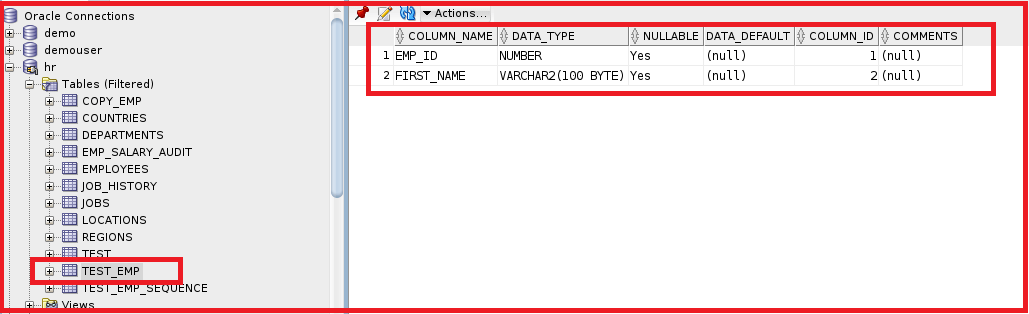So, the table is present. Let us check the triggers on the table TEST_EMP.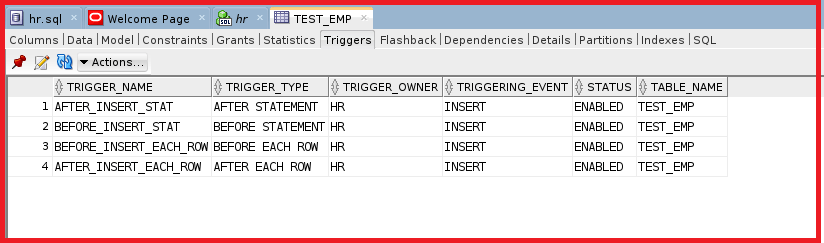So, we can see there are four triggers present in the table TEST_EMP.

##### Step 4: Test the Triggers

Let us go ahead and test the triggers by inserting data into the table TEST_EMP. Let us execute the following insert statement.

insert into test_emp values (1,’abc’);

The table TEST_EMP is having four triggers. When we execute the insert statement all four triggers will be executed. We have to make sure which trigger is executed first. We have created a sequence to make sure to check which trigger is executed first. In each trigger, we have mentioned the sequence number in order to get the order of triggers.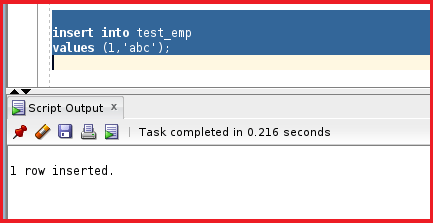So, the row is inserted. Let us check the TEST_EMP_SEQUENCE and see if the trigger is working fine or not. We can query the table TEST_EMP_SEQUENCE to check the table by executing the following SELECT Statement.

select * from test_emp_sequence order by seq;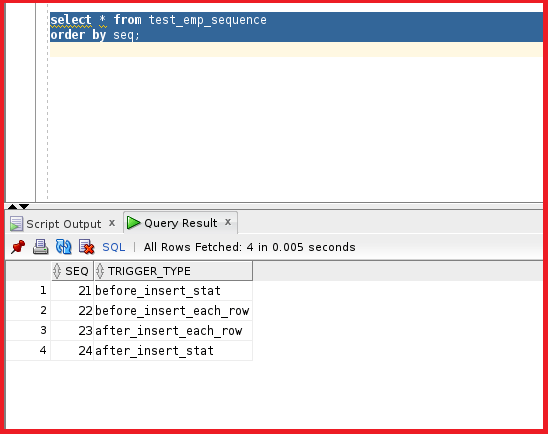You can see the sequence is created. The sequence is 21, 22, 23, and 24 and the order is the same. So, now let us try to run the insert statement for multiple rows and see how the sequence is created by executing the following SQL Statement.

```Insert into test_emp
Select employee_id, first_name from employees
Where department_id=20;
```

Here, we are inserting the employee_id and first name from the employee with department_id as 20 to the table TEST_EMP. Let us run this statement and see how it works.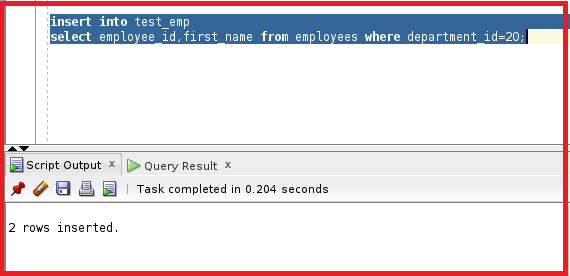We can see two rows are inserted. Let us check the sequence table and see how the sequence is generated by executing the following SQL Statement.

select * from test_emp_sequence order by seq;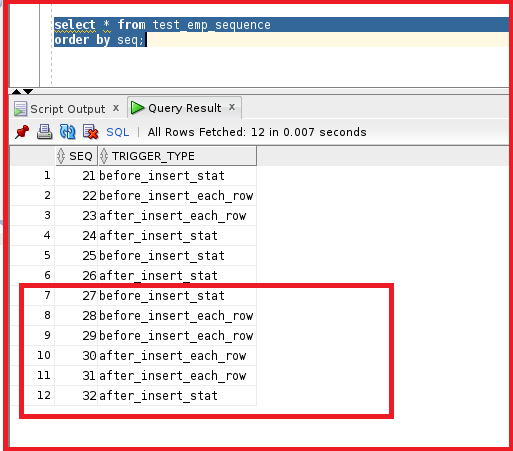From the output, you can see the sequence is generated. The sequences from 27 to 32 are generated during the insert operation. First, the before_insert_stat is generated and then before_insert_each_row is generated because there are two rows so, the trigger is executed twice. Then the after_insert_each_row is generated twice because of two rows in the table TEST_EMP and then the after_insert_stat is generated. So, all four triggers are executed in the same order as we discussed first. So, we have learned about the firing sequence of triggers in oracle.

In the next article, I am going to discuss Trigger Options in Oracle with Examples. Here, in this article, I try to explain Trigger Firing Sequence in Oracle with Examples. I hope you enjoy this Trigger Firing Sequence in the Oracle article.